Question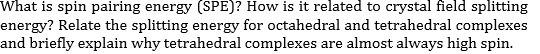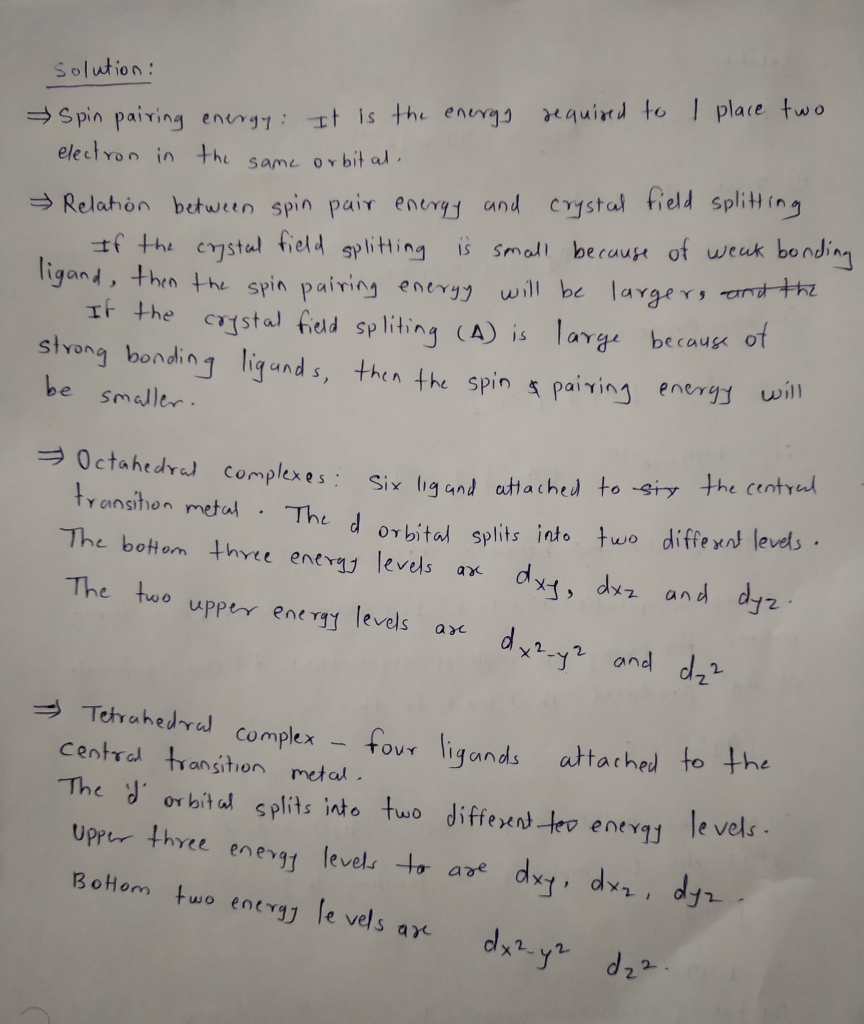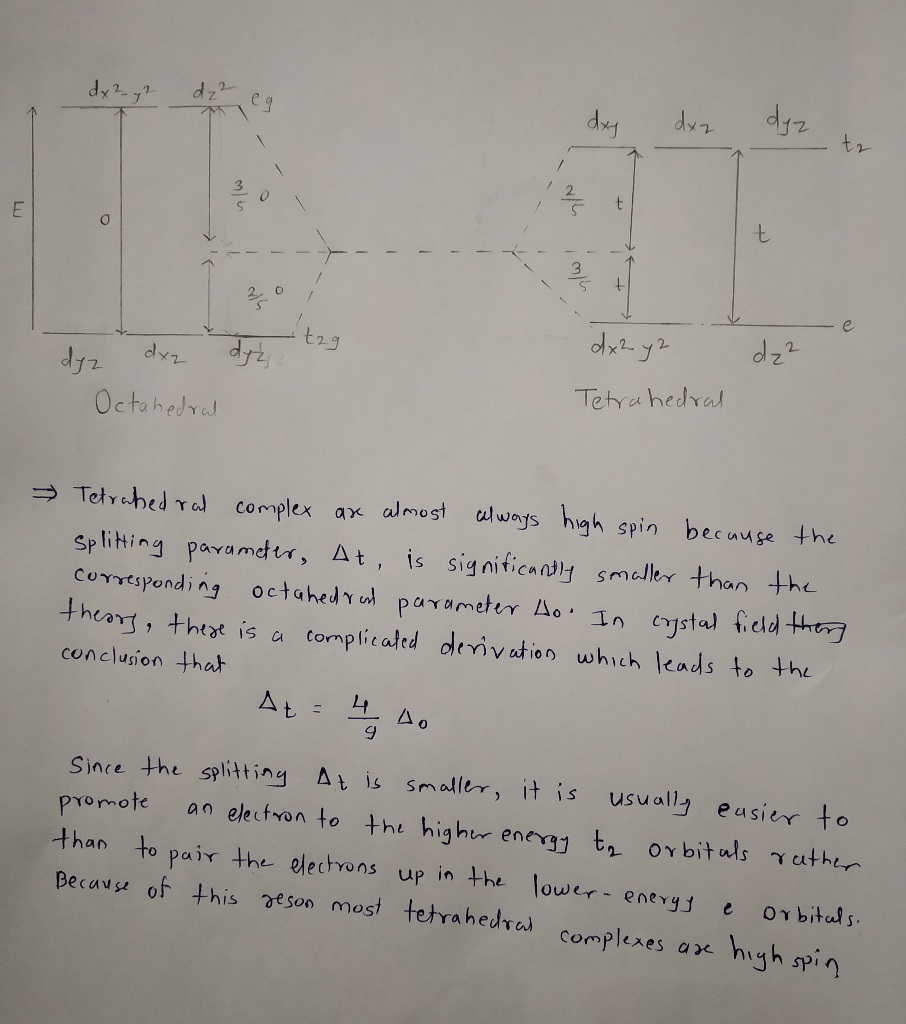#### Earn Coins

Coins can be redeemed for fabulous gifts.

Similar Homework Help Questions
• ### What is spin pairing energy (SPE)? How is it related to crystal field splitting energy? Relate...

What is spin pairing energy (SPE)? How is it related to crystal field splitting energy? Relate the splitting energy for octahedral and tetrahedral complexes and briefly explain why tetrahedral complexes are almost always high spin.

• ### Q2 (5 points) Part A (2 marks) Briefly explain how variations in ligand field stabilization energy...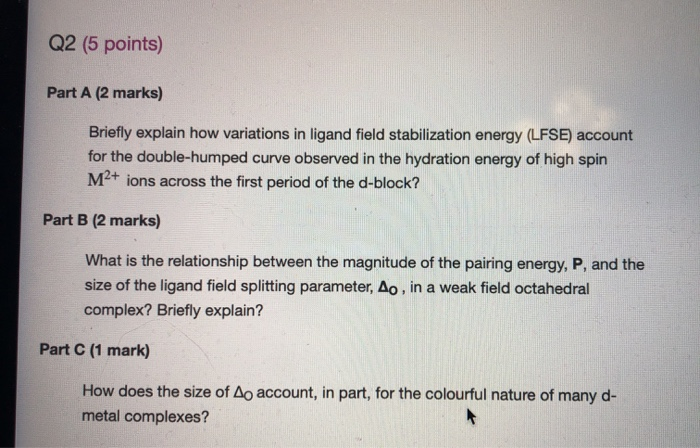Q2 (5 points) Part A (2 marks) Briefly explain how variations in ligand field stabilization energy (LFSE) account for the double-humped curve observed in the hydration energy of high spin M2+ ions across the first period of the d-block? Part B (2 marks) What is the relationship between the magnitude of the pairing energy, P, and the size of the ligand field splitting parameter, Ao , in a weak field octahedral complex? Briefly explain? Part C (1 mark) How does...

• ### Match the appropriate octahedral crystal-field splitting diagram with the given spin state and metal ion. Metal...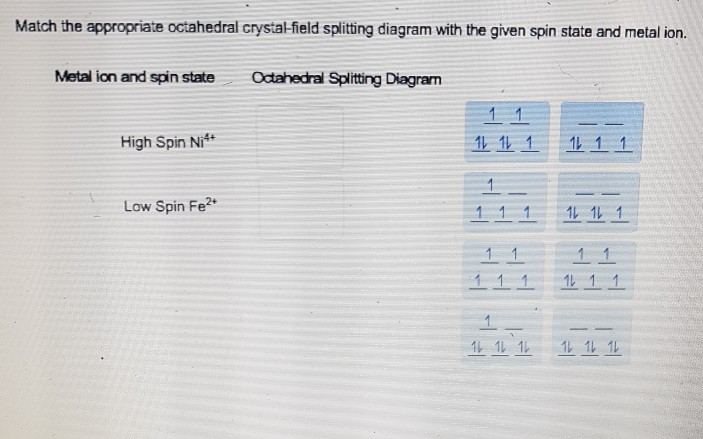Match the appropriate octahedral crystal-field splitting diagram with the given spin state and metal ion. Metal ion and spin state Otahedral Splitting Diagram 4+ High Spin Ni Low Spin Fe

• ### Help with this question please! Match the appropriate octahedral crystal-field splitting diagram with the given spin...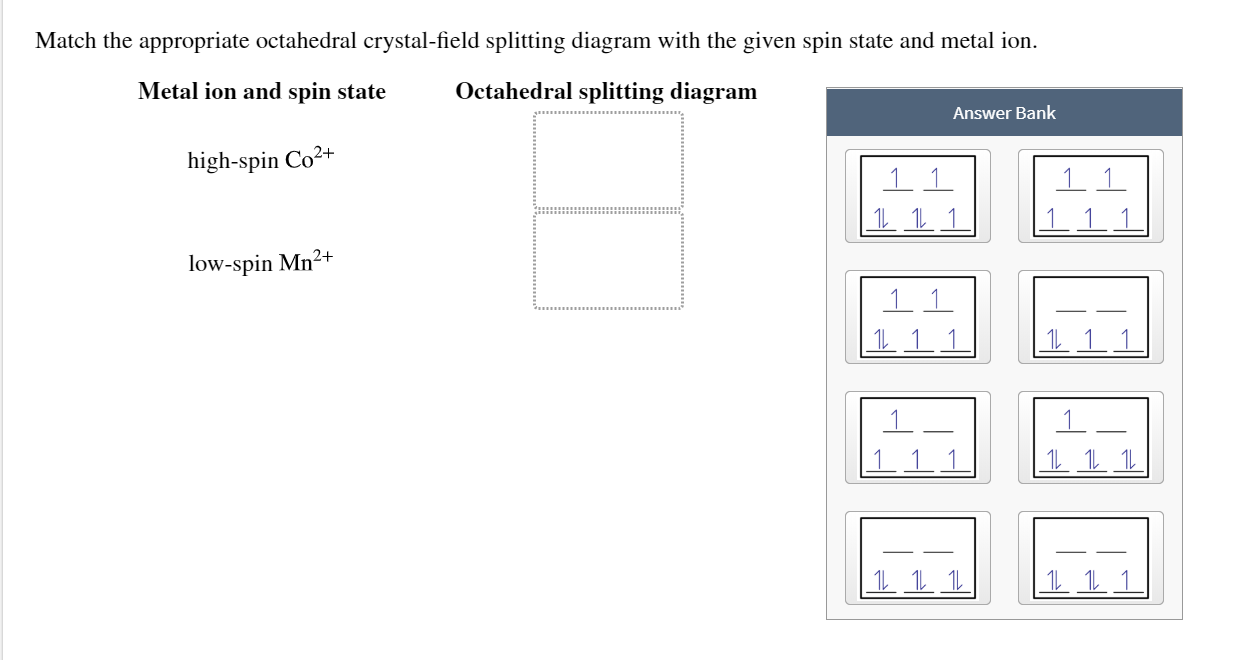Help with this question please! Match the appropriate octahedral crystal-field splitting diagram with the given spin state and metal ion. Metal ion and spin state Octahedral splitting diagram Answer Bank high-spin Co2+ 1 1 1 1 1 low-spin Mn2+ | 11 1 1 1 11 1 1 1 1

• ### ligand field will cause splitting of energy levels of 3d orbitals of the central metal ion.............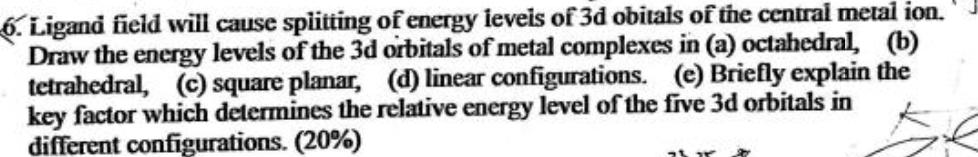ligand field will cause splitting of energy levels of 3d orbitals of the central metal ion.......... splitting of energy ieveis of 3d obitals of the central metal ion. 6. Ligand field will cause Draw the energy levels of the 3d ortbitals of metal complexes in (a) octahedral, (b) tetrahedral, (c) square planar, (d) linear configurations. (e) Briefly explain the key factor which determines the relative energy level of the five 3d orbitals in different configurations. (20 % ) splitting of...

• ### Match the appropriate octahedral crystal-field splitting diagram with the given spin state and metal ion Metal...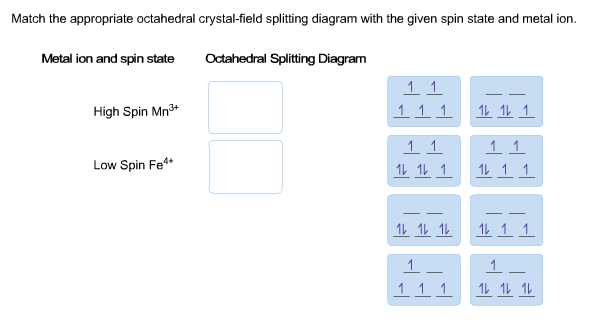Match the appropriate octahedral crystal-field splitting diagram with the given spin state and metal ion Metal ion and spin state Octahedral Splitting Diagram High Spin Mn3 111 1L111 Low Spin Fe" 1レ1レ1レ 1レ1 1 111 1レ 111レ

• ### Match the appropriate octahedral crystal-field splitting diagram with the given spin state and metal ion. Metal...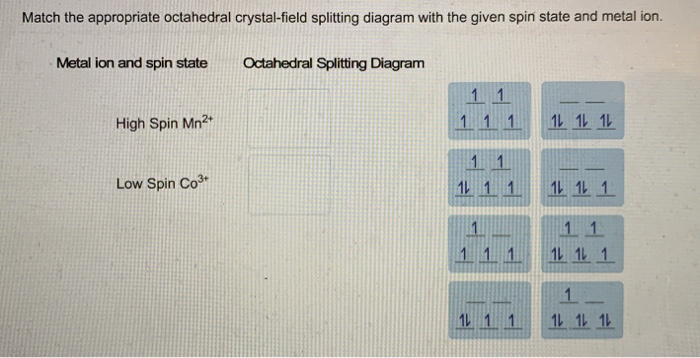Match the appropriate octahedral crystal-field splitting diagram with the given spin state and metal ion. Metal ion and spin state Octahedral Splitting Diagram High Spin Mn2+ 1 1 1 1L 1L 1L Low Spin Co3+ 1L 1 1 11 11 1 1 1 11 11 1 1 1 11 1 1 1L 1L 1L

• ### Match the appropriate octahedral crystal-field splitting diagram with the given spin state and metal ion. Metal...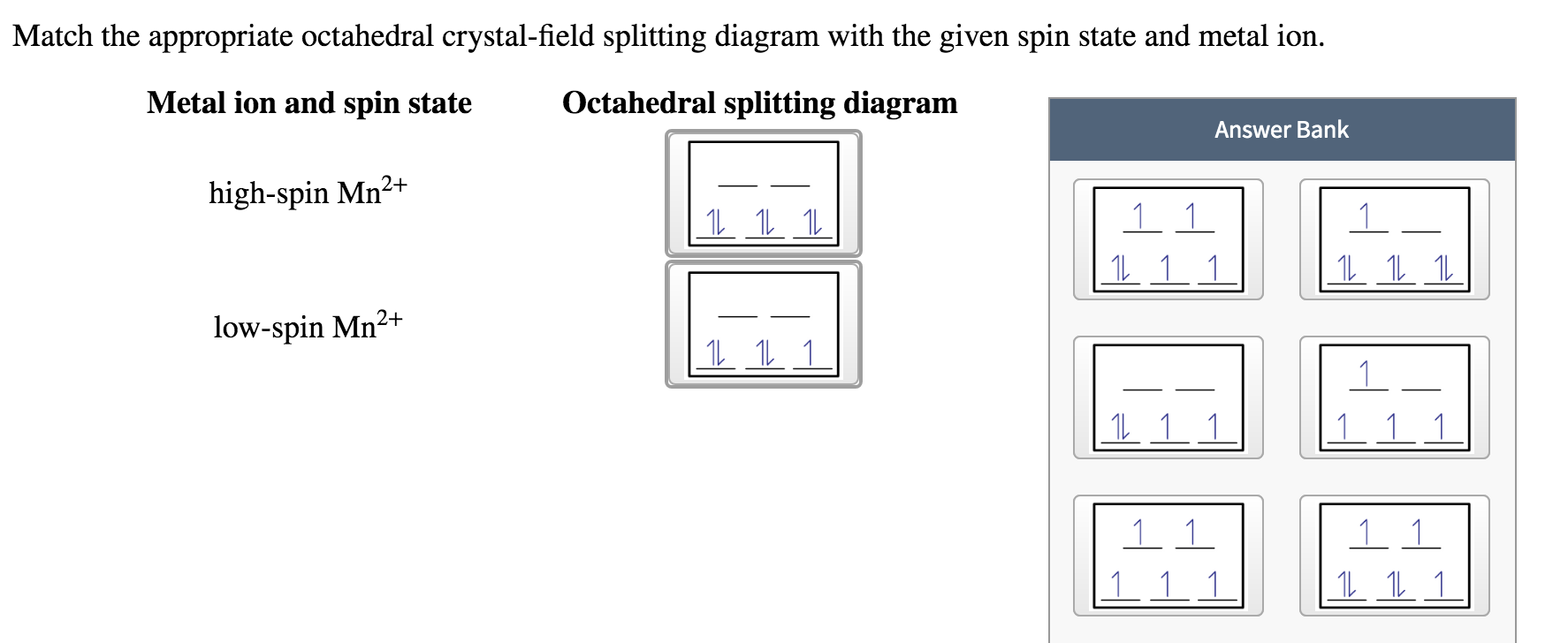Match the appropriate octahedral crystal-field splitting diagram with the given spin state and metal ion. Metal ion and spin state Octahedral splitting diagram Answer Bank high-spin Mn2+ 1 1 1 1 1 1 1 1 1. 11 11 11 low-spin Mn2+ 1L 1L 1 1 1 1 1 1 1 11 1 1 1 1 1

• ### Calculate the crystal field stabilization energy valiue for the complex Cr(NH)J*. The crystal field splitting energy...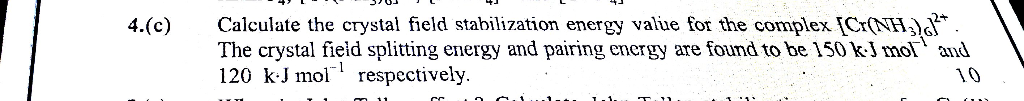Calculate the crystal field stabilization energy valiue for the complex Cr(NH)J*. The crystal field splitting energy and pairing energy are found to be 150 kJ mol and 120 kJ mol respectively. 4.(c) 10 cc. Calculate the crystal field stabilization energy valiue for the complex Cr(NH)J*. The crystal field splitting energy and pairing energy are found to be 150 kJ mol and 120 kJ mol respectively. 4.(c) 10 cc.

• ### Crystal Field Question. I understand that octahedral shapes have larger splitting energies then in tetrahedral complexes....Crystal Field Question. I understand that octahedral shapes have larger splitting energies then in tetrahedral complexes. And it would take more energy to split more electrons. So would the order be from Highest to Lowest: B>A>C where A, B, C are the compounds listed in their respective orders. Between Ni(H20)s2+ ; Ni(en)32+; and lowest and why? Cu(CN)42- which has highest splitting and which has